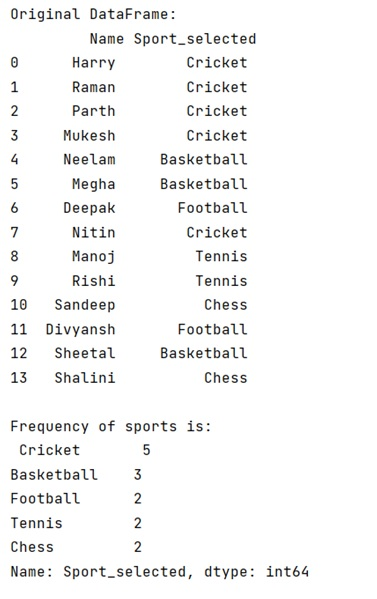# Count the frequency that a value occurs in a DataFrame column

Given a DataFrame, we need to count the frequency values in a particular column.
Submitted by Pranit Sharma, on April 28, 2022

In pandas, we can count the frequency of a particular column using DataFrame.value_count() method.

## pandas.DataFrame.value_counts() Method

This method is used to calculate the frequency of all values in a column separately. It takes the column name inside it as a parameter and returns a series of all the values with their respective frequencies. The important point is the that the returned series is descending in nature i.e., the value having highest frequency would be the first element of the series.

Syntax:

```DataFrame.value_counts(
subset=None,
normalize=False,
sort=True,
ascending=False,
dropna=True
)

# or
DataFrame.value_counts(["col_name"])
```

To work with Python Pandas, we need to import the pandas library. Below is the syntax,

```import pandas as pd
```

Let us understand with the help of an example.

```# Importing pandas package
import pandas as pd

# Creating a dictionary
dict = {
'Name':['Harry','Raman','Parth','Mukesh','Neelam','Megha','Deepak','Nitin','Manoj','Rishi','Sandeep','Divyansh','Sheetal','Shalini'],
}

# Creating a DataFrame
df=pd.DataFrame(dict)

# Display original DataFrame
print("Original DataFrame:\n",df,"\n")

# Counting frequency of column named Sport_selected
frequency_of_sports = df['Sport_selected'].value_counts()

# Display all the frequencies
print("Frequency of sports is:\n",frequency_of_sports,"\n")
```

Output:Languages: » C » C++ » C++ STL » Java » Data Structure » C#.Net » Android » Kotlin » SQL
Web Technologies: » PHP » Python » JavaScript » CSS » Ajax » Node.js » Web programming/HTML
Solved programs: » C » C++ » DS » Java » C#
Aptitude que. & ans.: » C » C++ » Java » DBMS
Interview que. & ans.: » C » Embedded C » Java » SEO » HR
CS Subjects: » CS Basics » O.S. » Networks » DBMS » Embedded Systems » Cloud Computing
» Machine learning » CS Organizations » Linux » DOS
More: » Articles » Puzzles » News/Updates